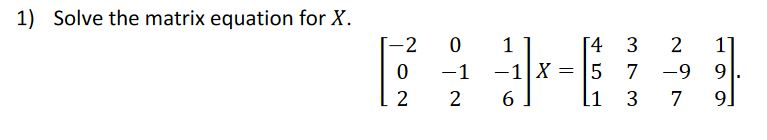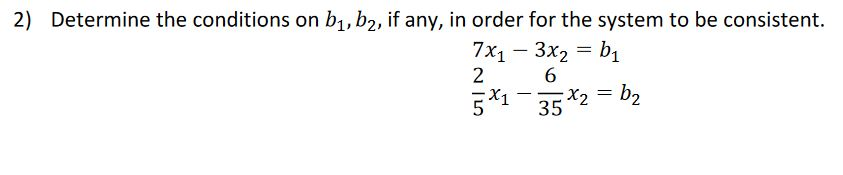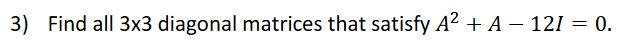Home / Answered Questions / Other / i-need-help-with-these-3-homework-problems-aw304

# (Solved): I Need Help With These 3 Homework Problems. ...

I need help with these 3 homework problems.1) Solve the matrix equation for X. 0 1 2 O -1 2 11 54 3 -1 X = 5 7 6) li 3 2 -9 7 1] 9. 9] 2) Determine the conditions on b1,b2, if any, in order for the system to be consistent. 7X1 - 3x2 = bi 2 6 x2 - x2 = b? 3) Find all 3x3 diagonal matrices that satisfy A2 + A - 121 = 0.

We have an Answer from Expert